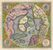# Survey123 - Leading Zeros for integer

775
2
04-23-2020 10:36 AMby
Regular Contributor

Hi guys,

I need to format a field to 4 digits in this way:

the user would input a number and

if 1 ----> the resulting number is 0001

if 12 ----> the resulting number is 0012

Is it possible to format this?

the field type is an integer and I put a length limit to 4.

thanks!

Tags (3)
1 Solution

Accepted SolutionsbyEsri Notable Contributor

This would do the job, assuming that your integer question has nu for name.

if(string-length(\${nu})=1,concat("000",\${nu}),if(string-length(\${nu})=2,concat("00",\${nu}),if(string-length(\${nu})=3,concat("0",\${nu}),\${nu})))

For example:

typenamelabelcalculationbind::esri:fieldType
integernuA numbernull
calculatenu_trailA number (trailing 0)if(string-length(\${nu})=1,concat("000",\${nu}),if(string-length(\${nu})=2,concat("00",\${nu}),if(string-length(\${nu})=3,concat("0",\${nu}),\${nu})))esriFieldTypeString
2 RepliesbyEsri Notable Contributor

This would do the job, assuming that your integer question has nu for name.

if(string-length(\${nu})=1,concat("000",\${nu}),if(string-length(\${nu})=2,concat("00",\${nu}),if(string-length(\${nu})=3,concat("0",\${nu}),\${nu})))

For example:

typenamelabelcalculationbind::esri:fieldType
integernuA numbernull
calculatenu_trailA number (trailing 0)if(string-length(\${nu})=1,concat("000",\${nu}),if(string-length(\${nu})=2,concat("00",\${nu}),if(string-length(\${nu})=3,concat("0",\${nu}),\${nu})))esriFieldTypeStringby
Regular Contributor

Sorry for the late answer: it is exactly what I was looking for!